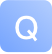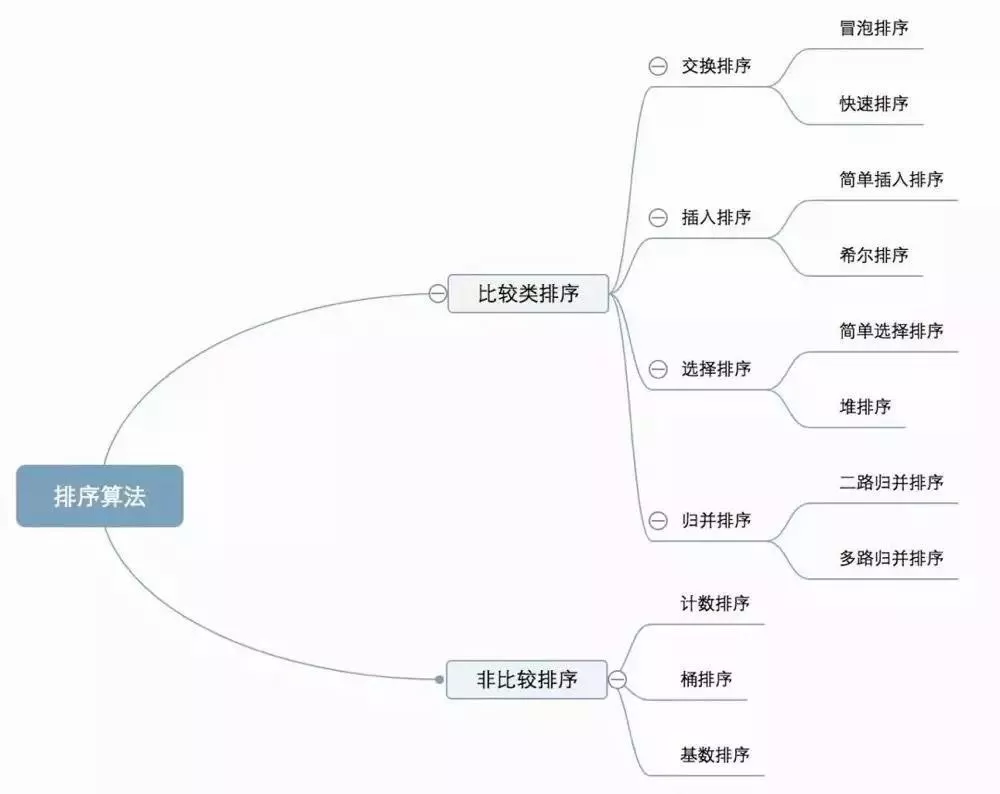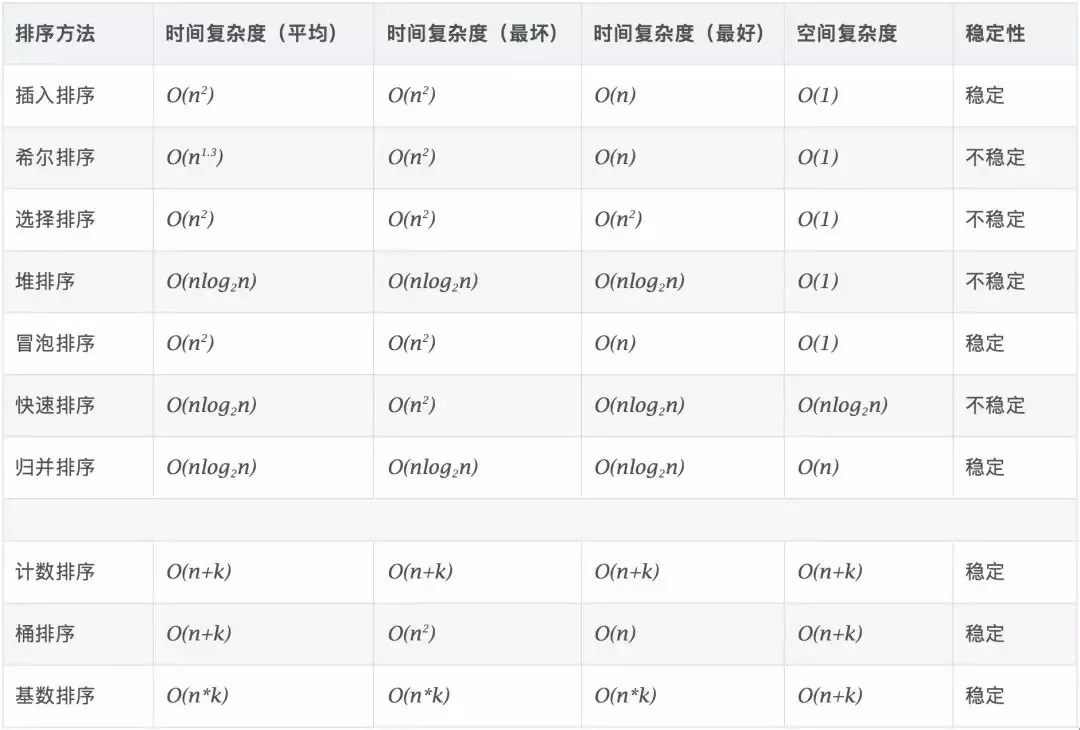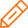# 十大经典排序算法最强总结(内含代码实现)-问答-阿里云开发者社区-阿里云## 十大经典排序算法最强总结(内含代码实现)

2020-01-09 14:44:55 6234 2

1、算法分类2、算法复杂度3、算法相关概念

4、具体说明

1、冒泡排序

1）算法步骤

3）什么时候最快

4）什么时候最慢

5）Java 代码实现

``````public class BubbleSort implements IArraySort {

@Override
public int[] sort(int[] sourceArray) throws Exception {
// 对 arr 进行拷贝，不改变参数内容
int[] arr = Arrays.copyOf(sourceArray, sourceArray.length);

for (int i = 1; i < arr.length; i++) {
// 设定一个标记，若为true，则表示此次循环没有进行交换，也就是待排序列已经有序，排序已经完成。
boolean flag = true;

for (int j = 0; j < arr.length - i; j++) {
if (arr[j] > arr[j + 1]) {
int tmp = arr[j];
arr[j] = arr[j + 1];
arr[j + 1] = tmp;

flag = false;
}
}

if (flag) {
break;
}
}
return arr;
}
}
``````

2、选择排序

1）算法步骤

3）Java 代码实现

``````public class SelectionSort implements IArraySort {

@Override
public int[] sort(int[] sourceArray) throws Exception {
int[] arr = Arrays.copyOf(sourceArray, sourceArray.length);

// 总共要经过 N-1 轮比较
for (int i = 0; i < arr.length - 1; i++) {
int min = i;

// 每轮需要比较的次数 N-i
for (int j = i + 1; j < arr.length; j++) {
if (arr[j] < arr[min]) {
// 记录目前能找到的最小值元素的下标
min = j;
}
}

// 将找到的最小值和i位置所在的值进行交换
if (i != min) {
int tmp = arr[i];
arr[i] = arr[min];
arr[min] = tmp;
}

}
return arr;
}
}
``````

3、插入排序

1）算法步骤

3）Java 代码实现

``````public class InsertSort implements IArraySort {

@Override
public int[] sort(int[] sourceArray) throws Exception {
// 对 arr 进行拷贝，不改变参数内容
int[] arr = Arrays.copyOf(sourceArray, sourceArray.length);

// 从下标为1的元素开始选择合适的位置插入，因为下标为0的只有一个元素，默认是有序的
for (int i = 1; i < arr.length; i++) {

// 记录要插入的数据
int tmp = arr[i];

// 从已经排序的序列最右边的开始比较，找到比其小的数
int j = i;
while (j > 0 && tmp < arr[j - 1]) {
arr[j] = arr[j - 1];
j--;
}

// 存在比其小的数，插入
if (j != i) {
arr[j] = tmp;
}

}
return arr;
}
}
``````

4、希尔排序

1）算法步骤

2）Java 代码实现

``````
public class ShellSort implements IArraySort {

@Override
public int[] sort(int[] sourceArray) throws Exception {
// 对 arr 进行拷贝，不改变参数内容
int[] arr = Arrays.copyOf(sourceArray, sourceArray.length);

int gap = 1;
while (gap < arr.length) {
gap = gap * 3 + 1;
}

while (gap > 0) {
for (int i = gap; i < arr.length; i++) {
int tmp = arr[i];
int j = i - gap;
while (j >= 0 && arr[j] > tmp) {
arr[j + gap] = arr[j];
j -= gap;
}
arr[j + gap] = tmp;
}
gap = (int) Math.floor(gap / 3);
}

return arr;
}
}
``````

5、归并排序

However, it is not possible to do so in JavaScript, as the recursion goes too deep for the language to handle.

1）算法步骤

3）Java 代码实现

``````public class MergeSort implements IArraySort {

@Override
public int[] sort(int[] sourceArray) throws Exception {
// 对 arr 进行拷贝，不改变参数内容
int[] arr = Arrays.copyOf(sourceArray, sourceArray.length);

if (arr.length < 2) {
return arr;
}
int middle = (int) Math.floor(arr.length / 2);

int[] left = Arrays.copyOfRange(arr, 0, middle);
int[] right = Arrays.copyOfRange(arr, middle, arr.length);

return merge(sort(left), sort(right));
}

protected int[] merge(int[] left, int[] right) {
int[] result = new int[left.length + right.length];
int i = 0;
while (left.length > 0 && right.length > 0) {
if (left <= right) {
result[i++] = left;
left = Arrays.copyOfRange(left, 1, left.length);
} else {
result[i++] = right;
right = Arrays.copyOfRange(right, 1, right.length);
}
}

while (left.length > 0) {
result[i++] = left;
left = Arrays.copyOfRange(left, 1, left.length);
}

while (right.length > 0) {
result[i++] = right;
right = Arrays.copyOfRange(right, 1, right.length);
}

return result;
}

}
``````

**6、快速排序 **

1）算法步骤

3）Java 代码实现

``````public class QuickSort implements IArraySort {

@Override
public int[] sort(int[] sourceArray) throws Exception {
// 对 arr 进行拷贝，不改变参数内容
int[] arr = Arrays.copyOf(sourceArray, sourceArray.length);

return quickSort(arr, 0, arr.length - 1);
}

private int[] quickSort(int[] arr, int left, int right) {
if (left < right) {
int partitionIndex = partition(arr, left, right);
quickSort(arr, left, partitionIndex - 1);
quickSort(arr, partitionIndex + 1, right);
}
return arr;
}

private int partition(int[] arr, int left, int right) {
// 设定基准值（pivot）
int pivot = left;
int index = pivot + 1;
for (int i = index; i <= right; i++) {
if (arr[i] < arr[pivot]) {
swap(arr, i, index);
index++;
}
}
swap(arr, pivot, index - 1);
return index - 1;
}

private void swap(int[] arr, int i, int j) {
int temp = arr[i];
arr[i] = arr[j];
arr[j] = temp;
}

}
``````

7、堆排序

1）算法步骤

3）Java 代码实现

``````public class HeapSort implements IArraySort {

@Override
public int[] sort(int[] sourceArray) throws Exception {
// 对 arr 进行拷贝，不改变参数内容
int[] arr = Arrays.copyOf(sourceArray, sourceArray.length);

int len = arr.length;

buildMaxHeap(arr, len);

for (int i = len - 1; i > 0; i--) {
swap(arr, 0, i);
len--;
heapify(arr, 0, len);
}
return arr;
}

private void buildMaxHeap(int[] arr, int len) {
for (int i = (int) Math.floor(len / 2); i >= 0; i--) {
heapify(arr, i, len);
}
}

private void heapify(int[] arr, int i, int len) {
int left = 2 * i + 1;
int right = 2 * i + 2;
int largest = i;

if (left < len && arr[left] > arr[largest]) {
largest = left;
}

if (right < len && arr[right] > arr[largest]) {
largest = right;
}

if (largest != i) {
swap(arr, i, largest);
heapify(arr, largest, len);
}
}

private void swap(int[] arr, int i, int j) {
int temp = arr[i];
arr[i] = arr[j];
arr[j] = temp;
}

}
``````

8、计数排序

2）Java 代码实现

``````
public class CountingSort implements IArraySort {

@Override
public int[] sort(int[] sourceArray) throws Exception {
// 对 arr 进行拷贝，不改变参数内容
int[] arr = Arrays.copyOf(sourceArray, sourceArray.length);

int maxValue = getMaxValue(arr);

return countingSort(arr, maxValue);
}

private int[] countingSort(int[] arr, int maxValue) {
int bucketLen = maxValue + 1;
int[] bucket = new int[bucketLen];

for (int value : arr) {
bucket[value]++;
}

int sortedIndex = 0;
for (int j = 0; j < bucketLen; j++) {
while (bucket[j] > 0) {
arr[sortedIndex++] = j;
bucket[j]--;
}
}
return arr;
}

private int getMaxValue(int[] arr) {
int maxValue = arr;
for (int value : arr) {
if (maxValue < value) {
maxValue = value;
}
}
return maxValue;
}

}
``````

9、桶排序

1）什么时候最快

2）什么时候最慢

3）Java 代码实现

``````public class BucketSort implements IArraySort {

private static final InsertSort insertSort = new InsertSort();

@Override
public int[] sort(int[] sourceArray) throws Exception {
// 对 arr 进行拷贝，不改变参数内容
int[] arr = Arrays.copyOf(sourceArray, sourceArray.length);

return bucketSort(arr, 5);
}

private int[] bucketSort(int[] arr, int bucketSize) throws Exception {
if (arr.length == 0) {
return arr;
}

int minValue = arr;
int maxValue = arr;
for (int value : arr) {
if (value < minValue) {
minValue = value;
} else if (value > maxValue) {
maxValue = value;
}
}

int bucketCount = (int) Math.floor((maxValue - minValue) / bucketSize) + 1;
int[][] buckets = new int[bucketCount];

// 利用映射函数将数据分配到各个桶中
for (int i = 0; i < arr.length; i++) {
int index = (int) Math.floor((arr[i] - minValue) / bucketSize);
buckets[index] = arrAppend(buckets[index], arr[i]);
}

int arrIndex = 0;
for (int[] bucket : buckets) {
if (bucket.length <= 0) {
continue;
}
// 对每个桶进行排序，这里使用了插入排序
bucket = insertSort.sort(bucket);
for (int value : bucket) {
arr[arrIndex++] = value;
}
}

return arr;
}

/**
* 自动扩容，并保存数据
*
* @param arr
* @param value
*/
private int[] arrAppend(int[] arr, int value) {
arr = Arrays.copyOf(arr, arr.length + 1);
arr[arr.length - 1] = value;
return arr;
}

}
``````

10、基数排序

1）基数排序 vs 计数排序 vs 桶排序

3）Java 代码实现

``````/**
* 基数排序
* 考虑负数的情况还可以参考：https://code.i-harness.com/zh-CN/q/e98fa9
*/
public class RadixSort implements IArraySort {

@Override
public int[] sort(int[] sourceArray) throws Exception {
// 对 arr 进行拷贝，不改变参数内容
int[] arr = Arrays.copyOf(sourceArray, sourceArray.length);

int maxDigit = getMaxDigit(arr);
}

/**
* 获取最高位数
*/
private int getMaxDigit(int[] arr) {
int maxValue = getMaxValue(arr);
return getNumLenght(maxValue);
}

private int getMaxValue(int[] arr) {
int maxValue = arr;
for (int value : arr) {
if (maxValue < value) {
maxValue = value;
}
}
return maxValue;
}

protected int getNumLenght(long num) {
if (num == 0) {
return 1;
}
int lenght = 0;
for (long temp = num; temp != 0; temp /= 10) {
lenght++;
}
return lenght;
}

private int[] radixSort(int[] arr, int maxDigit) {
int mod = 10;
int dev = 1;

for (int i = 0; i < maxDigit; i++, dev *= 10, mod *= 10) {
// 考虑负数的情况，这里扩展一倍队列数，其中 [0-9]对应负数，[10-19]对应正数 (bucket + 10)
int[][] counter = new int[mod * 2];

for (int j = 0; j < arr.length; j++) {
int bucket = ((arr[j] % mod) / dev) + mod;
counter[bucket] = arrayAppend(counter[bucket], arr[j]);
}

int pos = 0;
for (int[] bucket : counter) {
for (int value : bucket) {
arr[pos++] = value;
}
}
}

return arr;
}

/**
* 自动扩容，并保存数据
*
* @param arr
* @param value
*/
private int[] arrayAppend(int[] arr, int value) {
arr = Arrays.copyOf(arr, arr.length + 1);
arr[arr.length - 1] = value;
return arr;
}
}
``````取消 提交回答

• 游客5sblnickduwac
2020-03-18 10:04:20

优秀

0 0
• 游客pklijor6gytpx
2020-01-09 14:46:15

加入阿里云钉钉群享福利：每周技术直播，定期群内有奖活动、大咖问答0 0添加回答

1

2022-06-30 10:48:06 133浏览量 回答数 1

1

2022-05-12 16:27:01 345浏览量 回答数 1

1

2021-04-30 17:02:16 411浏览量 回答数 1

1

2020-06-08 16:33:08 575浏览量 回答数 1

1

2020-02-12 19:51:08 1263浏览量 回答数 1

2

oss 资源包购买后服务器快照备份还在收费

2018-10-09 11:49:22 782浏览量 回答数 2

1

2018-07-21 17:07:21 1677浏览量 回答数 1

1

2018-07-19 11:34:41 1598浏览量 回答数 1

1

2018-07-17 19:29:59 1642浏览量 回答数 1

2

2016-04-20 22:26:23 5056浏览量 回答数 2
0

2554